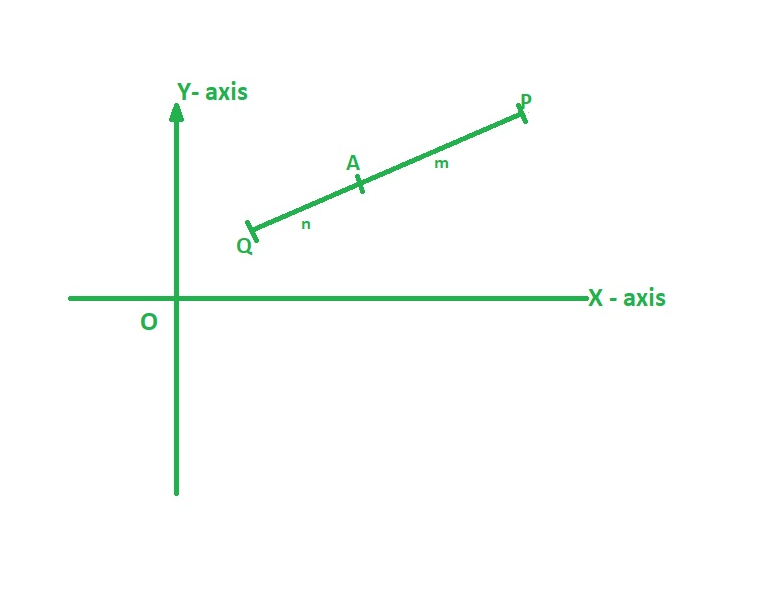Open in App
Not now

# Find the ratio in which the line joining the points (- 3, 10) and (6, -8) is divided by the point (- 1, 6)?

• Last Updated : 01 Oct, 2021

We are familiar with plotting points on a graph in a plane. Points are usually used to locate a point called as coordinates. Coordinate Geometry is a branch of study that deals with coordinates, the distance between coordinates, Midpoint between two coordinates, a point that divides a line in a particular ratio……, etc. Coordinate Geometry relates Algebra and Geometry by using equations, lines. Position of a point that is coordinates are represented using an ordered pair of numbers where the first number represents x-axis of the plane while other represents y-axis.

### Section Formula

Section formula is used to find the point that divides a line segment in a ratio that is known and coordinates of line segments are known. If a line segment PQ on a plane where P(x1, y1) and Q(x2, y2) is divided by a point A(x, y) in ratio m:n as shown in the figure, then point A can be found using section formula. We can also find the point of the line segment by taking ratio as 1:1.A (x, y) = ((mx2 + nx1) / (m + n), (my2 + ny1) / (m + n))

If the point that divides the line segment externally then the external section is

A (x, y) = ((mx2 – nx1) / (m – n), (my2 – ny1) / (m – n))

### Find the ratio in which the line joining the points (- 3, 10) and (6, -8) is divided by the point (- 1, 6)?

Solution:

Given,
Points of line segment: (-3,10) (6,-8)
Point dividing line segment: (-1,6)
Since, the point is dividing the line segment internally we use section formula:
A (x, y) = ((mx2 + nx1) / (m + n), (my2 + ny1) / (m + n))
By substituting values as x = -1, y = 6, x1 = -3, x2 = 6 we get
=> -1 = (6m + (-3n))/(m + n)
=> -1(m + n) = 6m – 3n
=> -m – n = 6m -3n
=> -m – 6m = n – 3n
=> -7m = -2n
=> m / n = 2/7
=> m : n = 2 : 7
Therefore, ratio in which point(-1, 6) divides the line segment joining (-3, 10), (6, -8) is 2:7

### Midpoint Formula

Midpoint is defined as the point that divides a line segment exactly into half that is in the same ratio we can derive the midpoint formula from the section formula. If point A(x, y) is dividing the line segment joining P(x1, y1) and Q (x2, y2 ) in ratio 1:1 then A is said to be the midpoint of the line PQ.

By using Section Formula:

A (x, y) = ((mx2 + nx1) / (m + n), (my2 + ny1) / (m + n))

since, m : n = 1 : 1

A (x, y) = (((1)x2 + (1)x1) / (1 + 1), ((1)y2 + (1)y1) / (1 + 1))

A (x, y) = ((x2 + x1) / 2, (y2 + y1) / 2)

### Sample Questions

Question 1: What is meant by Point of Trisection?

Solution:

Point of Trisection is two points on line segment where the line is divided into 3 equal parts.
If a point P,Q is a point of trisection for line segment AB then AP : PQ : QB = 1 : 1 : 1

Question 2: Find coordinates of a point that divides the line segment joining points A (2, 1) and B (2, 7) in the ratio 1:2?

Solution:

Given,
Points of line segment : (2,1) (2,7)
ratio in which line segment is divided is 1 : 2
Since, the point is dividing the line segment internally we use section formula:
A (x, y) = ((mx2 + nx1) / (m + n), (my2 + ny1) / (m + n))
By substituting values as m = 1, n = 2, x1 = 2, x2 = 2, y1 = 1, y2 = 7 we get
A (x, y) = ((1*2 + 2*2) / (1 + 2), (1*7 + 2*1) / (1 + 2))
A (x, y) = (6/3, 9/3)
A (x, y) = (2, 3)
Therefore, point that divides the line segment joining points A (2, 1) and B (2, 7) is (2, 3)

Question 3: Find the Midpoint of the line segment joining A (5, 3) and B (3, 7)?

Solution:

Given,
Points of line segment : (5,3) (3,7)
Mid point formula:
A (x, y) = ((x2 + x1) / 2, (y2 + y1) / 2)
A (x, y) = ((5 + 3) / 2, (3 + 7) / 2)
A (x, y) = (8 / 2, 10 / 2)
A (x, y) = (4, 5)
Therefore, Midpoint of the line segment joining A (5, 3) and B (3, 7) is (4, 5)

Question 4: What is the ratio in which the joining of points (2, 1) and (2, 7) is divided by the point (2, 3)?

Solution:

Given,
Points of line segment: (2,1) (2,7)
Point dividing line segment: (2,3)
Since, the point is dividing the line segment internally we use section formula:
A (x, y) = ((mx2 + nx1) / (m + n), (my2 + ny1) / (m + n))
By substituting values as x = 2, y = 3, y1 = 1, y2 = 7 we get
=> 3 = (7m + n)/(m + n)
=> 3(m + n) = 7m + n
=> 3m + 3n = 7m + n
=> -7m + 3m = n – 3n
=> -4m = -2n
=> m / n = 2/4
=> m : n = 1 : 2
Therefore, ratio in which point(2,3) divides the line segment joining (2,1),(2,7) is 1:2

My Personal Notes arrow_drop_up